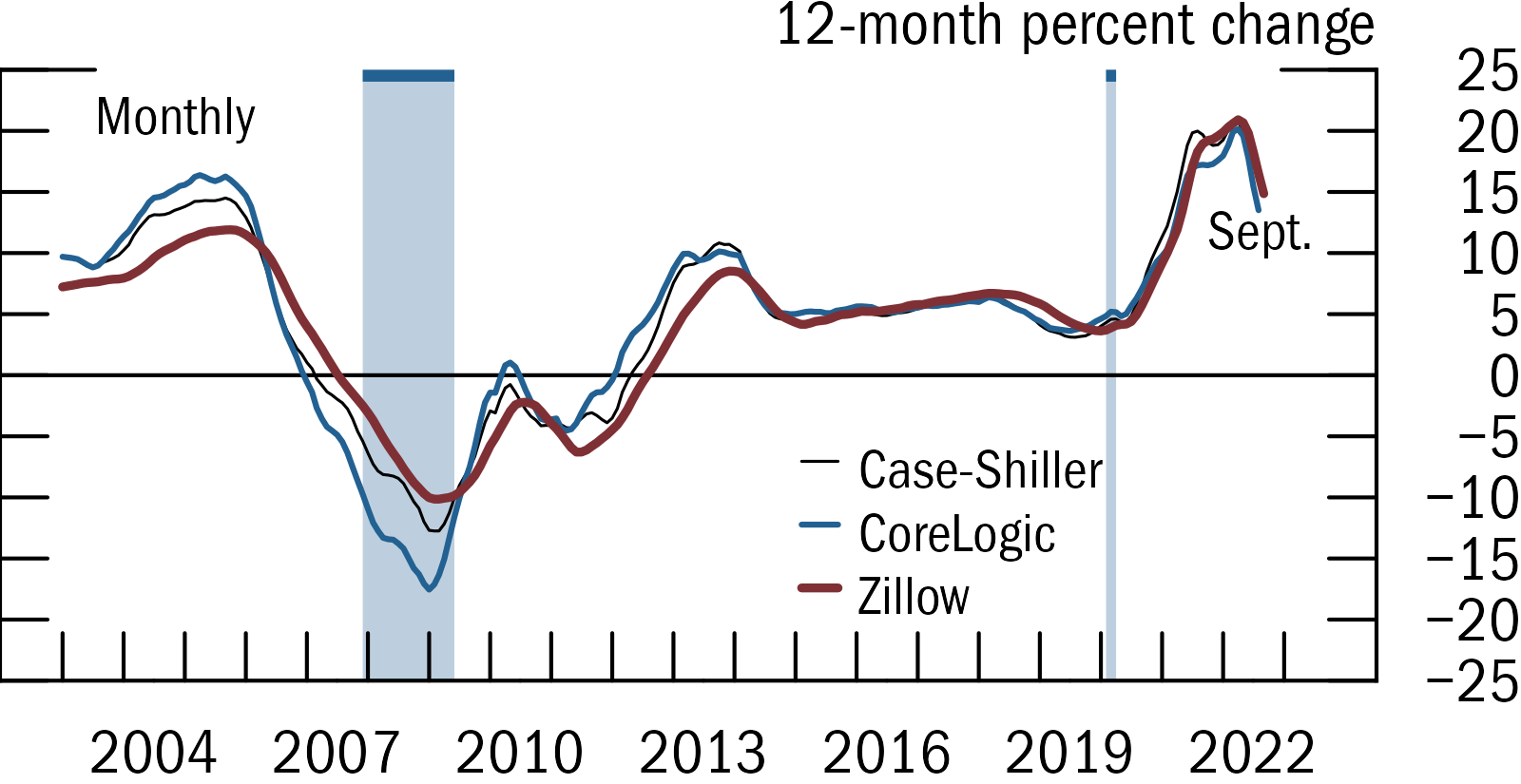This is an R package that allows users to replicate Fed-style plots (e.g. FSR, Tealbook) within ggplot. It contains a theme and several ggplot functions to customize and export figures.

It’s currently a work-in-progress (version 0.2), with planned improvements including:

1. Wrappers around options, so they are less verbose (e.g. scale_* below).
2. Documentation and website.
3. Better automated placement of legend and text labels.
4. Better testing of different OSes and chart types.
5. Document that fonts and other options can be changed through options()

## Quick example

(Also see here for a self-contained script)

library(fedplot)

ggplot(plotdata, aes(x = Date, y = value, group=source)) +
geom_recessions()
geom_hline(yintercept = 0, linewidth = getOption("fedplot.linewidth_adj"), linejoin = "mitre", lineend = "round") +
geom_line(aes(color=source, linewidth=source), na.rm = T, linejoin = "mitre", lineend = "round") +
labs(y = "12-month percent change") +
scale_x_date(minor_breaks=seq(from=as.Date("2003-01-01"), to=as.Date("2023-01-01"), by="1 years"),
breaks=seq(from=as.Date("2004-06-30"), to=as.Date("2023-06-30"), by="3 years"),
date_labels="%Y",
expand=expansion(mult=.10)) +
scale_y_continuous(sec.axis = dup_axis(),
breaks = seq(-25, 25, by=5),
limits = c(-25, 25),
expand = expansion(mult=0),
labels = scales::label_number(style_negative = "minus")) +
annotate_last_date(nudge_y = -3, nudge_x = 0) +
theme_fed(legend_position = c(.55, .5))

save_plot('my-figure', size='narrow', extension='png')## Installation

To install or update fedplot:

## Install current version from GitHub
devtools::install_github("fedplot/fedplot", build_vignettes=FALSE)

## Then load the package as you would any other
library(fedplot)

## To-Do

1. Add documentation and pkgdown website
2. Convert line.R into a vignette.
3. Add examples for bar charts
4. Add wrappers to the geom_hline (maybe set as an option to theme_fed), to the scale_* functions, and to geom_line (so it uses the group aes into its color and linewidth aes, plus the other options).

### Possible improvements

1. Ensure that certain geometries (e.g. recession bars) are always in the background, independently on the order in which they were added. This can be achieved with e.g. move_layers from the gginnards package.

## Acknowledgments

• Recession bars as well as some design ideas are based on the great cmapplot library mantained by the Chicago Metropolitan Agency for Planning.